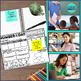# 3rd GRADE NUMBER OF THE DAY | NUMBER SENSE | MATH MORNING WORK | MATH HOMEWORKSubject
Resource Type
File Type

PDF

(2 MB|183 pages)
Standards
Also included in:
1. This bundle includes a collection of math materials to use with your 3rd grade students. Inside you'll find an entire year of spiral review homework/morning work, 3 assessements for every standard, vocabulary cards and so much more!Please take a look at the previews for each of the resources in this
\$116.50
\$65.24
Save \$51.26
• Product Description
• StandardsNEW
Number of the Day 3rd Grade

Today's Number / Number of the Day 3rd Grade Common Core Daily Math Review

This product contains 183 printable pages to make it easy to review important math skills on a regular basis.

I also have a version available for 2nd and 4th grade if you need to differentiate within your classroom.

I created the Today’s Number series to use in my own classroom as a spiral review for my third graders. I include one page each week as part of my Math Workshop / Guided Math Rotations.

You may also find it useful to use the pages as homework or when you have a sub. I keep one copy on hand as a “go to back up activity” in the event that I am unexpectedly called out of the classroom or if I’ve planned a lesson involving technology only to find that said technology is choosing not to work at that specific moment.

INTRODUCING THE TODAY’S NUMBER ACTIVITY:
I found it best to introduce the page slowly. Instead of just passing them out and having them get started I first modeled how to fill in the boxes (only a few at a time) using my projector over the course of several days.

Next, I walked the students through the form again in small groups.

Finally, I keep a completed model available for them to look at if needed, but they complete the page independently (or sometimes with a partner).

THE BACK OF TODAY’S NUMBER:
Originally I had my early finishers use the number of the day to create equations that equal that number. However, I found that some wrote too large and disorganized. I also noticed that some students were simply writing equations such as, “99+1=100, 98+2=100, 97+3=100, etc” All were accurate, but certainly not stretching their minds.

It was for those reasons that I created a form. It has two columns to help organize their work. Also, it includes a box for “rules.” One rule that is included every. single. day is my “no patterns rule” which prevents them from the list above. Other rules require them to challenge themselves a bit. Some examples include:
Each equation MUST include a + and a -.
Each equation needs to be written using only even numbers.
Each equation must include 4 addends.

Because the activity is open-ended and can literally go on forever, I have them keep their paper in their folder to use when other activities are completed during the week and collect them on Friday.
Fluently multiply and divide within 100, using strategies such as the relationship between multiplication and division (e.g., knowing that 8 × 5 = 40, one knows 40 ÷ 5 = 8) or properties of operations. By the end of Grade 3, know from memory all products of two one-digit numbers.
Understand division as an unknown-factor problem. For example, find 32 ÷ 8 by finding the number that makes 32 when multiplied by 8.
Apply properties of operations as strategies to multiply and divide. Examples: If 6 × 4 = 24 is known, then 4 × 6 = 24 is also known. (Commutative property of multiplication.) 3 × 5 × 2 can be found by 3 × 5 = 15, then 15 × 2 = 30, or by 5 × 2 = 10, then 3 × 10 = 30. (Associative property of multiplication.) Knowing that 8 × 5 = 40 and 8 × 2 = 16, one can find 8 × 7 as 8 × (5 + 2) = (8 × 5) + (8 × 2) = 40 + 16 = 56. (Distributive property.)
Determine the unknown whole number in a multiplication or division equation relating three whole numbers. For example, determine the unknown number that makes the equation true in each of the equations 8 × ? = 48, 5 = __ ÷ 3, 6 × 6 = ?.
Use multiplication and division within 100 to solve word problems in situations involving equal groups, arrays, and measurement quantities, e.g., by using drawings and equations with a symbol for the unknown number to represent the problem.
Total Pages
183 pages
N/A
Teaching Duration
1 Year
Report this Resource to TpT
Reported resources will be reviewed by our team. Report this resource to let us know if this resource violates TpT’s content guidelines.\$4.00
List Price:
\$5.00
You Save:
\$1.00